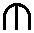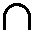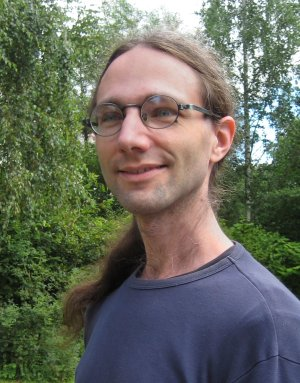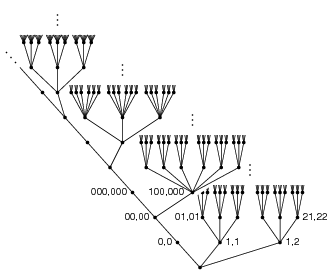Immi
:= Immanuel HalupczokChair of Algebra and Number TheoryGRK 2240: Algebro-geometric Methods in Algebra, Arithmetic and Topology Mathematical InstituteFaculty of Mathematics and Natural SciencesHHU Düsseldorf

 Lehrstuhl für Algebra und Zahlentheorie Mathematisches Institut Heinrich-Heine-Universität Düsseldorf Gebäude 25.22 Universitätsstr. 1 40225 Düsseldorf Germany Office: 25.22.03.46 Phone: +49 211 81-13189 E-mail:Math
EnglishA tree associated to the cusp curve X3 = Y2 in Z3; see Trees of definable sets in Zp for details.

My main research area are geometric and arithmetic questions in henselian valued fields, which I consider using model theoretic methods. Typical examples of henselian valued fields are the p-adic numbers and the field of formal Laurent series with coefficients in some other field. More precisely, I am mainly working on the following two topics:

• Singularities and stratifications: I proved the existence of a strong notion of stratifications in henselian valued fields. These stratifications in particular induce classical Whitney stratifications over the reals and the complex numbers.
• Motivic integration and its applications to representation theory of reductive groups over local fields: Motivic integration makes it possible to compute integrals uniformly in all local fields; this can be applied to make representation theory of reductive groups over local fields uniform in the field.

Other topics I worked on:

• Representation theory (of real reductive groups), symmetric varieties
• Model theory of pseudo-algebraically closed fields
• Combinatorics
• Polyomino achievement games
• Zero-sum problems in finite abelian groups
• Covering codes